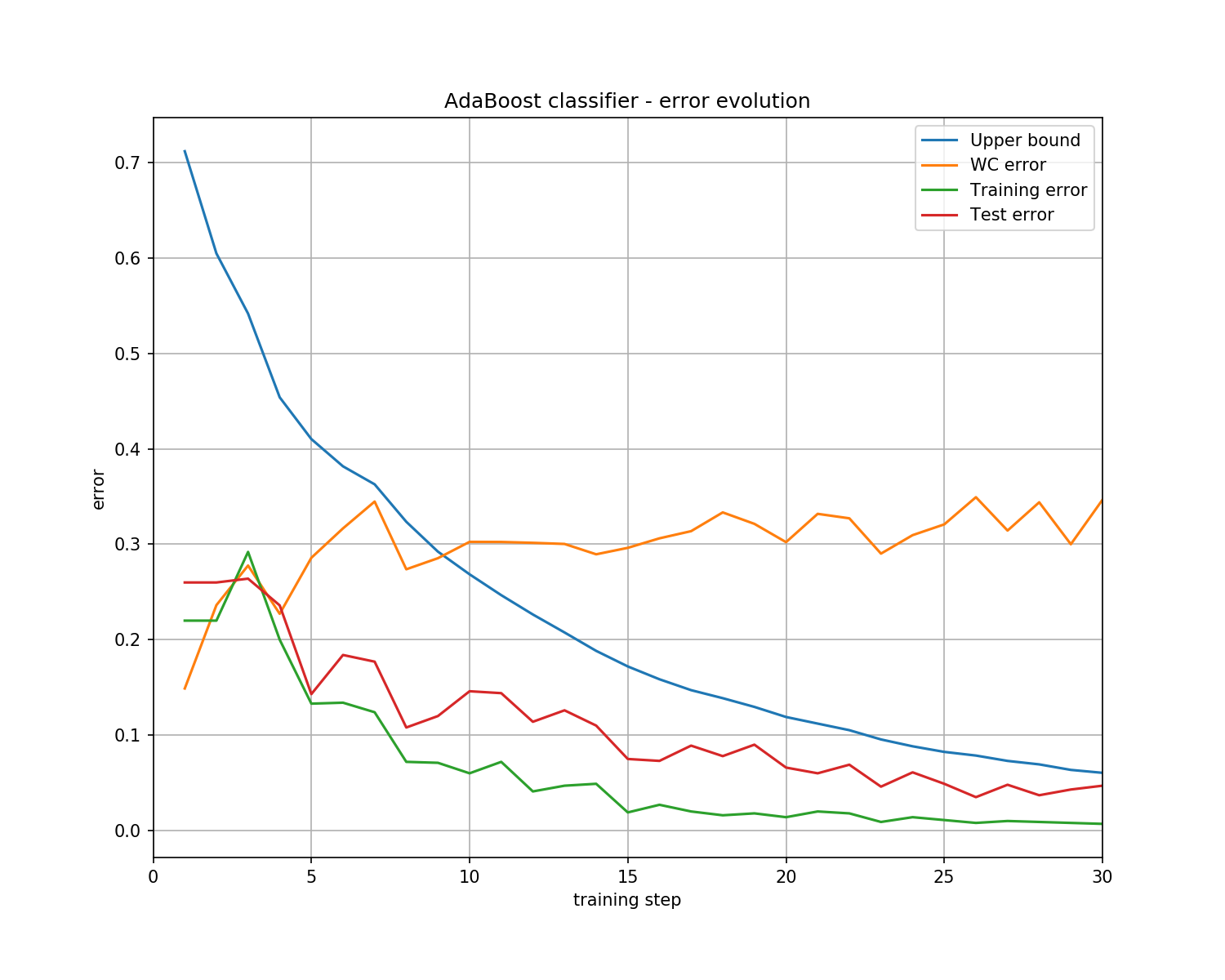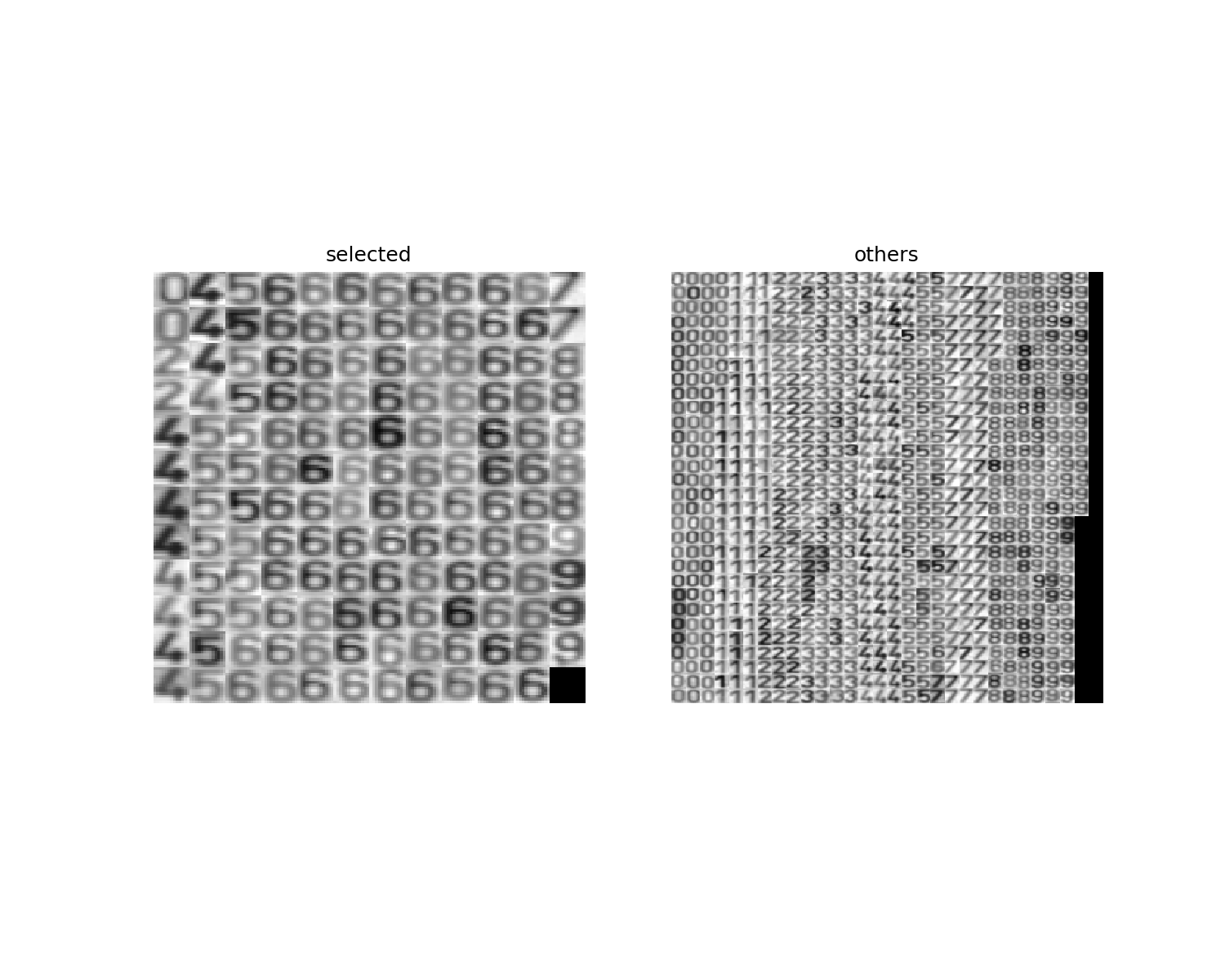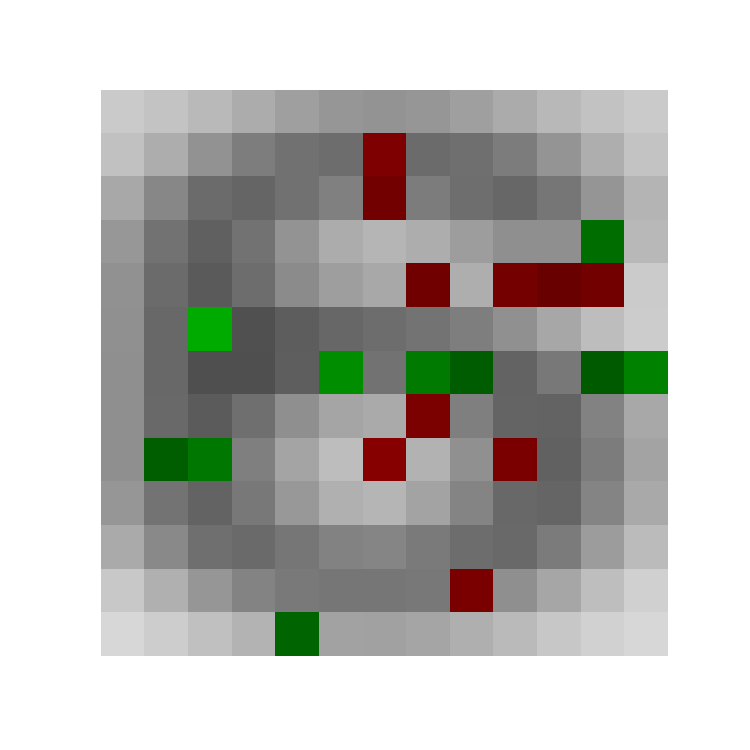Warning

At this week's labs we will implement the AdaBoost algorithm. The AdaBoost algorithm takes a class of “weak” classifiers and boosts their performance by combining them together to a “strong” classifier with adaptive weights assigned to each of the weak classifiers (AdaBoost stands for “adaptive boosting”). This way even relatively simple classifiers can be boosted to practically interesting accuracy levels.

In Adaboost, the classification function $H(x)$ has the form $$H(x) = \mbox{sign}(F(x))$$ $$F(x)=\sum^T_{t=1}\alpha_t h_t(x)$$ where $h_t(x)\;: \mathcal{X} \to \{-1, +1\}$ are the selected weak classifiers and $\alpha_t$ are the mixing weights.

The training algorithm itself is relatively simple:

Input: Training data $\{(x_1,y_1),\dotsc,(x_n,y_n)\}$; $x_i \in \mathcal{X}, y_i \in (-1, 1)$, number of iterations $T$, a set of weak classifiers $\mathcal{H}$

Initialise training data weights: $D_1(i) = 1/n$ for $i = 1,\dots,n$.
For $t = 1$ to $T$:

1. Select weak classifier with lowest weighted error:
$h_t = \underset{h_j \in \mathcal{H}}{\operatorname{argmin}} \epsilon_j$, where $$\epsilon_j=\sum^n_{i=1} D_t(i)\mathbf{1}[y_i \neq h_j(x_i)]$$ and $\mathbf{1}[\cdot]$ is the indicator function, which is 1 if the condition is satisfied, and 0 otherwise.
2. if $\epsilon_t \geq 0.5$ then stop
3. set weak classifier weight: $\alpha_t = \frac{1}{2}\log(\frac{1-\epsilon_t}{\epsilon_t})$
4. For $i=1,\dots,n$:
update $D_{t+1}(i) = \frac{1}{Z_t}D_t(i)\exp(-\alpha_t y_i h_t(x_i))$,
where $Z(t)$ is the normalisation factor that ensures that $D_{t+1}$ is a distribution (i.e. sums up to one)

Output: “Strong” classifier $H(x)=\mbox{sign}(F(x)) = \mbox{sign}(\sum^T_{t=1}\alpha_t h_t(x))$

In this assignment, we will use the AdaBoost learning algorithm to train a digit classifier. The input to the algorithm will be a set of 13×13 grayscale images of digits and their corresponding labels (0-9). We will select one of the digits as positive class ($y_i = +1$) and the rest of the images will form the negative part of the training set ($y_i = -1$). The task is then to distinguish the selected digit from the rest of the digits.

We are also free to choose the set of weak classifiers, $\mathcal{H}$. Ideally, we would like them to be discriminative enough for the given task but they do not necessarily need to be “too strong”. In our case, possible weak classifiers include local binary patterns (LBP), we may compute various histogram based features, use some edge-based features,… We may use single threshold decisions, build decision trees or use some form of regression. Note, that this is the strength of the AdaBoost algorithm – you are free to combine various weak classifiers and the algorithm determines which ones are useful for the task. Our dataset is rather small and simple, so to see the effects of weak classifiers combination into a stronger one, we will keep the weak classifiers intentionally rather simple.

Each weak classifier $h_{r,c}(I; \theta, p)\in\mathcal{H}$ is based on intensity value of one pixel and is parametrised by four numbers: its coordinates in the image $I$, row $r$ and column $c$, and by an intensity threshold $\theta\in R$ and a parity flag $p \in \{+1, -1\}$ indicating whether the positive class is above or below the threshold. Each such weak classifier takes the image intensity at position $(r, c)$, compares it with the threshold $\theta$ and returns the class using the parity: $$h_{r,c}(I; \theta, p) = \mbox{sign}(p * (I(r,c) - \theta))$$ Note, that we have 13×13=169 weak classifiers which are further parametrised by the threshold and parity. This gives us large variety of weak classifiers while keeping them rather weak and simple (much stronger weak classifiers could be easily used if we consider the relations between pixels, like the above mentioned LBPs). When searching for the best weak classifier in step 1 of the AdaBoost learning algorithm we are thus searching over $\theta$ and $p$ parameters of each of the 169 “template” classifiers and select the one with the lowest weighted error.

## Information for development in Python

To fulfil this assignment, you need to submit these files (all packed in a single .zip file) into the upload system:

• answers.txt - answers to the Assignment Questions
• adaboost.ipynb - a script for data initialisation, calling of the implemented functions and plotting of their results (for your convenience, will not be checked)
• adaboost.py - containing the following implemented methods
• adaboost - a function which implements the AdaBoost algorithm
• adaboost_classify - a function which implements the strong classifier classification
• compute_error - a function for computing the evolution of training and test errors
• error_evolution.png, classification.png, weak_classifiers.png - images specified in the tasks

Use template of the assignment. When preparing a zip file for the upload system, do not include any directories, the files have to be in the zip file root.

1. Choose one digit as a positive class.

2. Complete the function strong_classifier, wc_errors, upper_bound = adaboost(X, y, num_steps) so that it implements the AdaBoost training.
The function accepts a training set, labels and the number of training steps. It should return: strong_classifier - the found strong classifier (see the comments in the code for its structure), wc_error - the weighted error of the selected weak classifier in each iteration, and upper_bound - the training error upper bound $\prod Z_t$.

To find the best weak classifier, use the function find_best_weak(X, y, D) which implements vectorised search for the optimal $\theta$ and $p$.

To reduce the effect of an unbalanced training set (1:9 positive to negative sample size ratio) which would put too much emphasis on the negative samples, initialise the weights $D_1$ such that the $\sum_{i: y_i=-1}D_1(i) = \sum_{i: y_i=+1}D_1(i) = 0.5$.

Hint 1: The update of weights $D_{t+1}$ could be easily implemented using vector notation. Try to understand what it does. If there is something unclear feel free to ask us.

Hint 2: The update of $D_{t+1}$ can be also written as (substituting in $\alpha_t$) $D_{t+1} = \frac{1}{Z_t} D_t \sqrt{\frac{\varepsilon_t}{1 - \varepsilon_t}}$ if $y_i = h_t(x_i)$ and $D_{t+1} = \frac{1}{Z_t} D_t \sqrt{\frac{1 - \varepsilon_t}{\varepsilon_t}}$ if $y_i \neq h_t(x_i)$.

Hint 3: To test the correctness of your solution, the curves and classification results below are for the digit 6 and you should get the same values.

X = np.array([[1, 1.5, 2.5, 3.0, 3.5, 5, 5.5, 6, 7., 8], [1, 4, 2, 8, 1, 4, 3, 5, 7, 11]])
y = np.array([1, -1, 1, -1, 1, -1, 1, -1, 1, -1])
strong_classifier, wc_error, upper_bound = adaboost(X, y, 3)
print('strong_classifier: ', strong_classifier, '\nwc_error: ', wc_error, '\nupper_bound: ', upper_bound)
# -> strong_classifier:  {'wc': array([{'idx': 1, 'theta': 3.5, 'parity': -1},
# ->                                   {'idx': 1, 'theta': 7.5, 'parity': -1},
# ->                                   {'idx': 0, 'theta': 6.5, 'parity': 1}], dtype=object),
# ->                      'alpha': array([1.09861229, 0.80471896, 0.80471896])}
# -> wc_error:    [0.1        0.16666667 0.16666667]
# -> upper_bound: [0.6        0.4472136  0.33333333]
3. Train a strong classifier for a digit of your choice with num_steps = 30.

4. Complete the function errors = compute_error(strong_classifier, X, y) which returns the evolution of the error on the set X over the training iterations (using longer and longer strong classifier). Apply it to computing both the training and test error evolution.

X = np.array([[1, 1.5, 2.5, 3.0, 3.5, 5, 5.5, 6, 7., 8], [1, 4, 2, 8, 1, 4, 3, 5, 7, 11]])
y = np.array([1, -1, 1, -1, 1, -1, 1, -1, 1, -1])
strong_classifier, wc_error, upper_bound = adaboost(X, y, 3)
trn_errors = compute_error(strong_classifier, X, y)
print('trn_error: ', trn_errors)
# -> trn_error:  [0.1 0.1 0. ]
5. Plot all the collected errors into one graph and save it as error_evolution.png6. Complete the function classify = adaboost_classify(strong_classifier, X) which uses the strong classifier to classify new data X.

7. Show the classification results and save the picture as classification.png.8. The adaboost.ipynb notebook contains also a simple visualisation of the found weak classifiers. Feel free to use it or even improve it to see what weak classifiers were chosen as useful for the given classification task. Save the result into weak_classifiers.png.## Assignment Questions

Fill the correct answers to your answers.txt file.

1. What stragegy does the AdaBoost algorithm use to select the weak classifier in each iteration?
• a) A classifier with the highest number of correctly classified training samples is selected
• b) A classifier with the lowest number of correctly classified training samples is selected
• c) A classifier with the highest weighted classification error on training samples is selected
• d) A classifier with the lowest weighted classification error on training samples is selected
2. How do the weights change in each iteration?
• a) Weights of correctly classified training samples are increased and weights of incorrectly classified samples are decreased
• b) Weights of incorrectly classified training samples are increased and weights of correctly classified samples are decreased
• c) Weights of weak classifiers which correctly classify training samples are increased and weights of weak classifiers which classify incorrectly are decreased
• d) Weights of weak classifiers which incorrectly classify training samples are increased and weights of weak classifiers which classify correctly are decreased
3. When does the AdaBoost algorithm stop? Select all that apply.
• a) When the error on the training set is zero
• b) When the error on the testing set is zero
• c) After given number of iterations $T$
• d) When error of the weak classifier is higher than 0.5
• e) When the same weak classifier is selected for the second time
4. How the $\alpha$ coefficients change in time?
• a) They tend to decrease as the later weak classifiers have to solve more difficult classification task and are thus less likely to be too good.
• b) They tend to increase as the later weak classifiers have to solve less difficult task and are thus more likely to be good.
• c) There is no obvious tendency and earlier weak classifiers could be as useful as later ones.
5. Which of the digit classes is the easiest to train (the least number of iterations needed to get zero test error)?